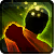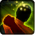# PummelConditions

Used by

## Related effects, buffs and debuffs

Please click on an effect below to view its details.

• [0s] [not displayed]Pummel

 Slot: Debuff Duration: 0s Tick rate: does not tick # occurrences: 0 Tags: 10284848162042239291
• On Apply

Perform the following actions:

• Weapon Damage
- Is Special Ability = (bool) false
- Ignore Dual Wield Modifier = (bool) false
- Unknown (609) = (bool) false
- Flurry Blows Min = (int) 3
- Flurry Blows Max = (int) 3
- Level Cap = (int) 0
- Threat Percent = (float) 0
- Standard Health Percent Max = (float) 0.03
- Standard Health Percent Min = (float) 0.03
- Amount Modifier Fixed Max = (float) 0
- Amount Modifier Fixed Min = (float) 0
- Amount Modifier Percent = (float) -1
- Coefficient = (float) 0
- Health Steal Percentage = (float) 0
- Slots = Int(5)
• On Apply
• [target override]

Perform the following actions:

• Add effect #2 to TARGET from TARGET
• [0s] [not displayed]Effect #2

 Slot: Debuff Duration: 0s Tick rate: does not tick # occurrences: 0 Conditions: Can only by called by other effects
• On Apply

Only when the following conditions are met:

• <
• If TARGET does not have 5 or more effects with the tag 15977813222904947394
AND
• If TARGET has an effect with the tag 15977813222904947394
>

Perform the following actions:

• Modify Charges
- Ability Spec = (int) 0
- Amount Max = (float) 1
- Amount Min = (float) 1
- Amount Percent = (float) 0
• On Apply

Only when the following conditions are met:

• <
• If TARGET does not have 5 or more effects with the tag 15977813222904947394
AND
• If TARGET has at least 2 effects with the tag 15977813222904947394
>

Perform the following actions:

• Modify Charges
- Ability Spec = (int) 0
- Amount Max = (float) 1
- Amount Min = (float) 1
- Amount Percent = (float) 0
• On Apply

Only when the following conditions are met:

• <
• If TARGET does not have 5 or more effects with the tag 15977813222904947394
AND
• If TARGET has at least 3 effects with the tag 15977813222904947394
>

Perform the following actions:

• Modify Charges
- Ability Spec = (int) 0
- Amount Max = (float) 1
- Amount Min = (float) 1
- Amount Percent = (float) 0
• On Apply

Only when the following conditions are met:

• <
• If TARGET does not have 5 or more effects with the tag 15977813222904947394
AND
• If TARGET has at least 4 effects with the tag 15977813222904947394
>

Perform the following actions:

• Modify Charges
- Ability Spec = (int) 0
- Amount Max = (float) 1
- Amount Min = (float) 1
- Amount Percent = (float) 0
• On Apply

Only when the following conditions are met:

• If TARGET has at least 5 effects with the tag 15977813222904947394

Perform the following actions:

• Modify Charges
- Ability Spec = (int) 0
- Amount Max = (float) 10
- Amount Min = (float) 10
- Amount Percent = (float) 0
• On Apply

Perform the following actions:

• Modify Charges
- Ability Spec = (int) 0
- Amount Max = (float) 1
- Amount Min = (float) 1
- Amount Percent = (float) 0